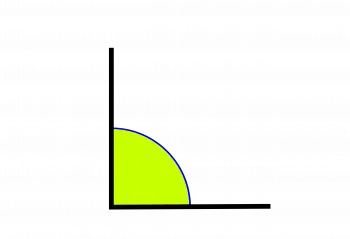# Right Angle: Definition, Measurement, Properties and ApplicationsThe right angle is one of the fundamental concepts in geometry and mathematics in general.

Its importance extends far beyond classrooms and the pages of textbooks, as it plays a crucial role in solving practical problems and understanding spatial relationships in the world around us.

In this article, we will take an in-depth look at what a right angle is, its properties, and how it is applied in various situations.

## Definition and Basic Properties

A right angle is a specific type of angle that measures exactly 90 degrees, making it a fundamental angle in Euclidean geometry. When represented graphically, a right angle looks like a perfectly square corner, similar to the corner of a sheet of paper or a box.

The following are some of the most important properties of right angles:

• Fixed Measurement: As mentioned above, a right angle always has a measure of 90 degrees, meaning it does not change in any geometric context.
• Perpendicular sides: In a right angle, the two sides that form it are perpendicular to each other. This means that they form a crossing of lines at 90 degrees, like the design of a cross.
• Addition of angles: Two right angles add up to 180 degrees, which is equivalent to a straight angle. A straight angle is a straight line, and half of a right angle is called an acute right angle and measures 45 degrees.

## Applications in Geometry

Right angles are an essential part of geometry and are used in a variety of geometric concepts and theorems.

Some of the most common uses include:

• Quadrilaterals: In the classification of quadrilaterals, right angles are an important characteristic. A rectangle, for example, has four right angles. In a square, all angles are right and measure 90 degrees.
• Right Triangles: Right triangles are a special type of triangle that contains a right angle. The Pythagorean theorem, which establishes the relationship between the sides of a right triangle, is one of the best-known results in geometry and is based on the presence of a right angle.
• Cartesian coordinates: In mathematics and analytical geometry, Cartesian coordinates use right angles to describe points on a plane. The x and y axes are perpendicular to each other, meaning they form right angles at each point of intersection.

## Applications in Everyday LifeRight angles also have applications in everyday life and in various disciplines:

• Architecture and construction: Right angles are essential in the construction of buildings and structures. Building foundations, walls, and corners are designed using right angles to ensure stability and alignment.
• Interior Design: In interior design, right angles are used to ensure that furniture and structures fit properly into a space. This includes the placement of windows, doors and furniture in a room.
• Surveying and cartography: Maps and topographical representations are based on right angles to show the precise spatial relationship between geographic features, such as streets, rivers, and property boundaries.
• Technology: In the manufacturing of electronic and mechanical devices, right angles are used to ensure precision and proper function of components.

## Conclusions

The right angle is a fundamental concept in geometry and has applications in many areas of everyday life.

Its fixed measurement of 90 degrees and the property that the sides are perpendicular make it an essential tool in solving geometric problems and representing spatial relationships.

Whether in building construction, interior planning or map making, right angles play a crucial role in our understanding and handling of the world around us.

Author:

Published: October 10, 2023
Last review: October 10, 2023